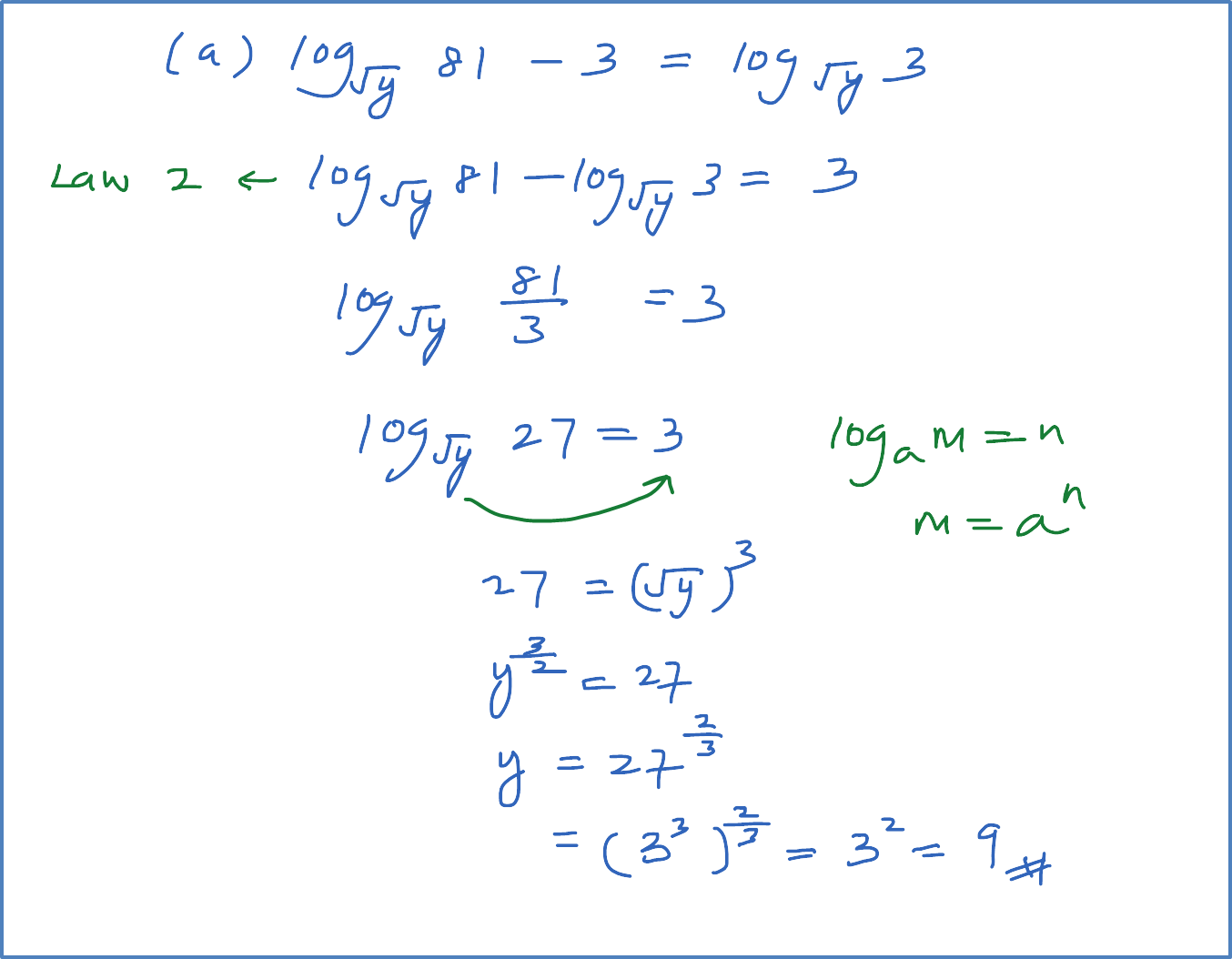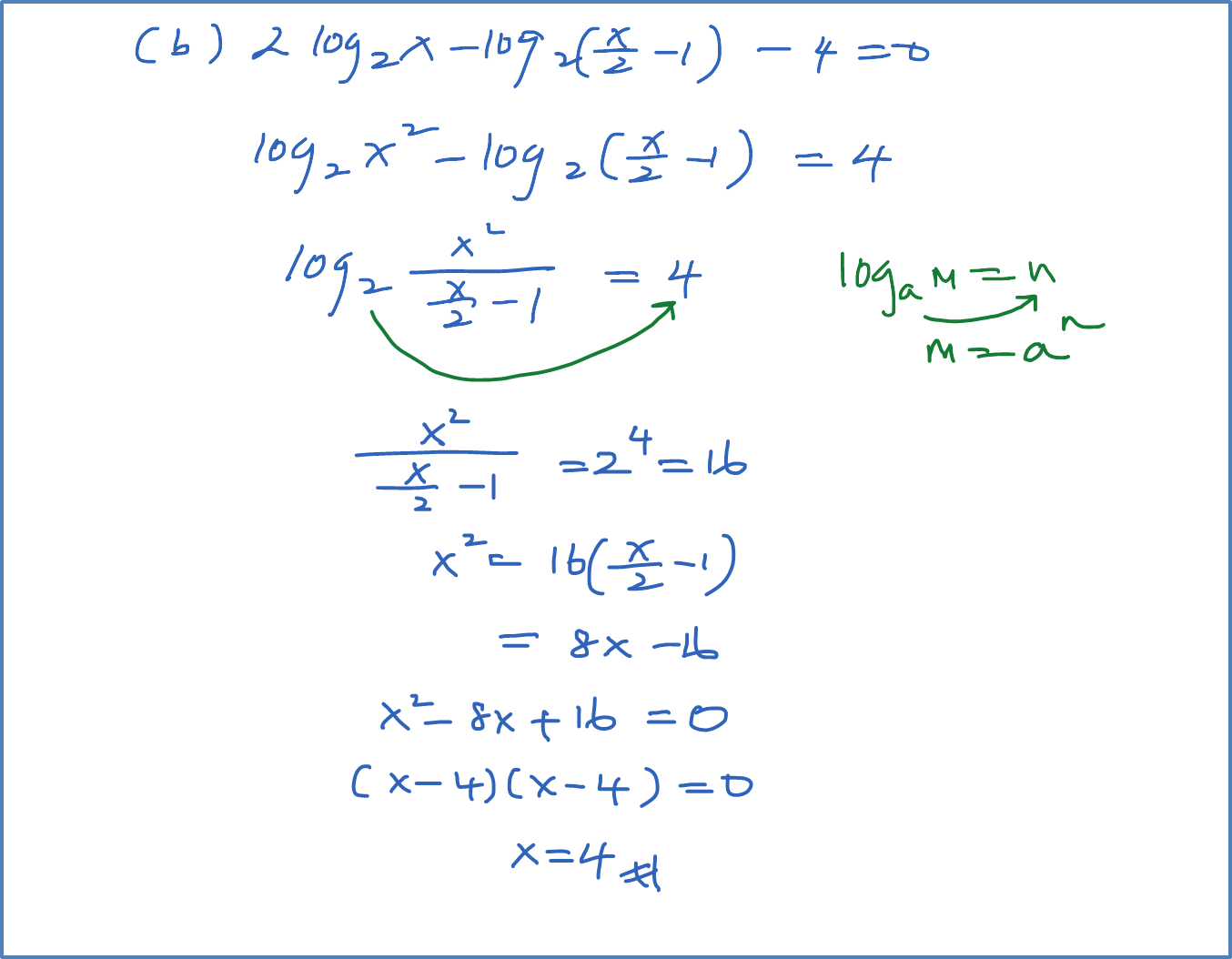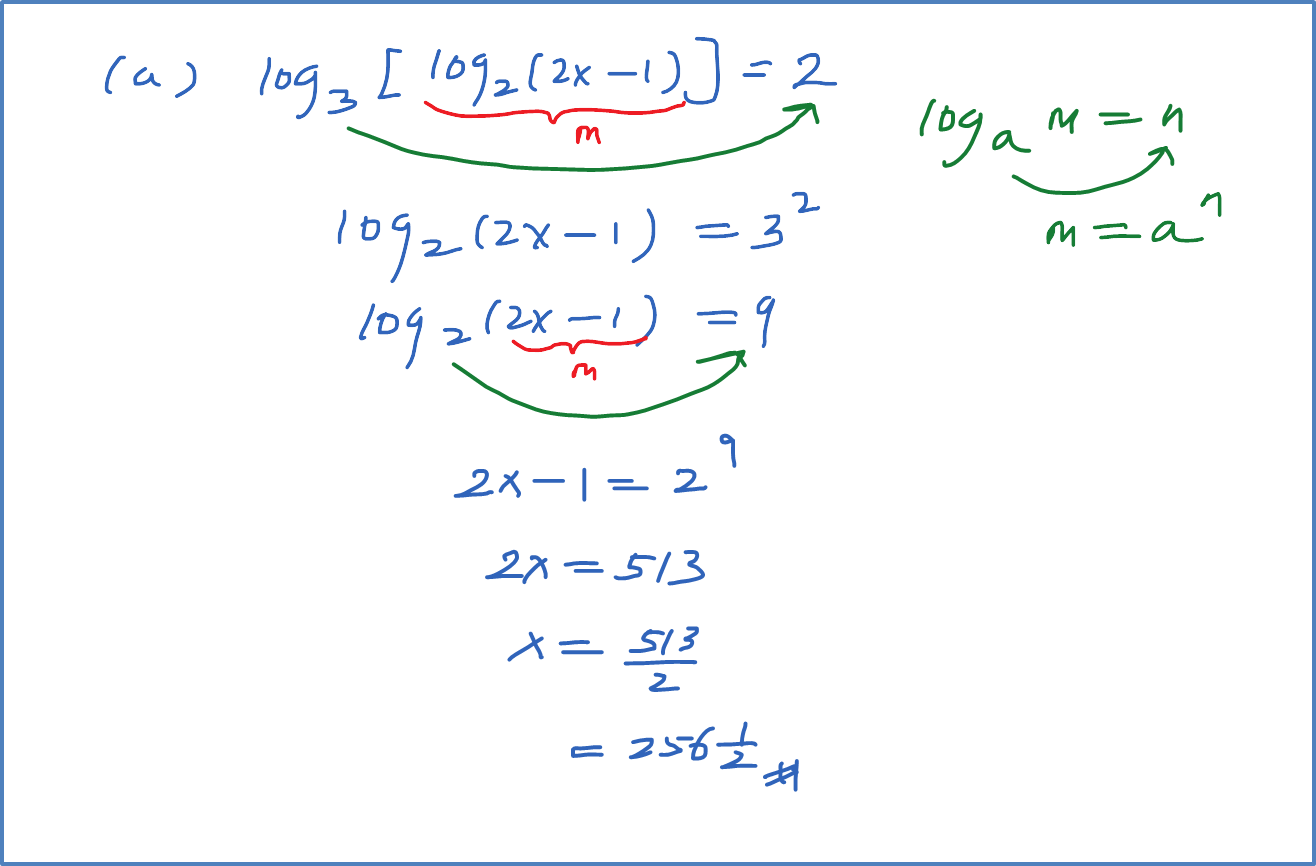\

# 4.4.2 Logarithms Equation – Example 2 & 3

Example 2
Solve the following equation:
(a) ${\mathrm{log}}_{\sqrt{y}}81-3={\mathrm{log}}_{\sqrt{y}}3$
(b) $2{\mathrm{log}}_{2}x-{\mathrm{log}}_{2}\left(\frac{x}{2}-1\right)-4=0$Example 3
Sole the following equation:
(a) ${\mathrm{log}}_{3}\left[{\mathrm{log}}_{2}\left(2x-1\right)\right]=2$
(b) ${\mathrm{log}}_{16}\left[{\mathrm{log}}_{2}\left(5x-4\right)\right]={\mathrm{log}}_{9}\sqrt{3}$